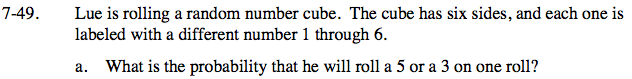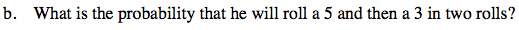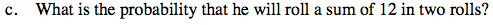Home > ACC7 > Chapter cc27 > Lesson cc27.1.4 > Problem7-49

7-49.
1. Lue is rolling a random number cube. The cube has six sides, and each one is labeled with a different number 1 through 6. Homework Help ✎

1. What is the probability that he will roll a 5 or a 3 on one roll?

2. What is the probability that he will roll a 5 and then a 3 in two rolls?

3. What is the probability that he will roll a sum of 12 in two rolls?Each number has an equal probability of being rolled. Since there are 6 total sides, what is the probability of getting any number?

Each number has a one-in-six probability of being rolled. Since we can roll either a 5 or a 3, we can add the probabilities of these two numbers together.

$\frac{1}{6}+\frac{1}{6}=?$There is a one-in-six probability of Lue rolling a 5 in the first roll, and there is a one-in-six probability of him rolling a 3 in the following roll. Since the order of the numbers rolled must be taken into account, we must multiply these two numbers together.

$\left(\frac{1}{6}\right)\left(\frac{1}{6}\right)=?$See part (b).